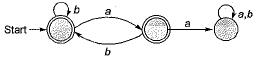Courses

# Finite Automata: Regular Languages - 1

## 10 Questions MCQ Test Question Bank for GATE Computer Science Engineering | Finite Automata: Regular Languages - 1

Description
This mock test of Finite Automata: Regular Languages - 1 for Computer Science Engineering (CSE) helps you for every Computer Science Engineering (CSE) entrance exam. This contains 10 Multiple Choice Questions for Computer Science Engineering (CSE) Finite Automata: Regular Languages - 1 (mcq) to study with solutions a complete question bank. The solved questions answers in this Finite Automata: Regular Languages - 1 quiz give you a good mix of easy questions and tough questions. Computer Science Engineering (CSE) students definitely take this Finite Automata: Regular Languages - 1 exercise for a better result in the exam. You can find other Finite Automata: Regular Languages - 1 extra questions, long questions & short questions for Computer Science Engineering (CSE) on EduRev as well by searching above.
QUESTION: 1

### Which of the following is false?

Solution:

For every NFA there exist an equivalent DFA and vice-versa. Power of both NFA and DFA for recognization of language is same.

QUESTION: 2

### Let r1 = ab*c*and r2 = (a*b+c)*and r3 = (a+b+c)*. Then which of the following is true

Solution:

ac ∈ L(r1), since we can take b* as ∈ and c* as c. ac ∈ L (r3), since (a + b + c ) * includes all combinations of a, b and c.
ac ∉ L(r2) since whenever (a * b + c)* is taken to include a, “a is always followed by b".
a*b = b, ab, aab, .... & so on.

QUESTION: 3

### Let ∑ = {a, b}, r1 = a(a + b)* and r2 = b(a + b)*. Which of the following is true?

Solution:

L(r1) is the set of all string starting with ‘a’.
L(r2) is the set of all starting with ‘b’.
Since any word belonging to ∑* either starts with “ a ” or starts with " b ” or is “∈ ” , therefore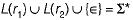QUESTION: 4

Which of the following statements are true?
(i) abcd ∈ L((b*a*)* (d*c*)*)
(ii) abcd ∈ L((d*c*b*a*)*)
(iii) abcd ∈ L((a*b*a*c*d*)*)

Solution:

Note that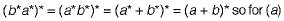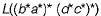gives all the combinations of a and b followed by all the combinations of c and d, for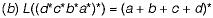will generate all strings with combination of a, b, c, b and d.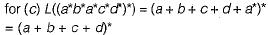QUESTION: 5

Which of the following are regular languages?
(i) The language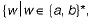w has an odd number of b’s}.
(ii) The language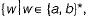w has an even number of b’s).
(iii) The language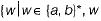has an even number of b’s and odd number of a’s).

Solution: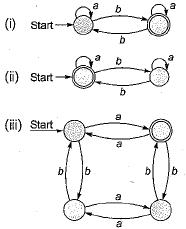As we can construct the DFA for the given languages, hence all of the given languages are regular.

QUESTION: 6

Which of the following regular expression corresponds to the language of all strings over the alphabet {a, b) that contains exactly two a’s
(i) aa
(ii) ab*a
(iii) b* ab*a

Solution:

All languages are accepting strings over the alphabet {a, b} that contains exactly two a’s but they are not accepting all strings like the first choice just accepts ‘aa’. The second choice can’t accept ‘baa’. The third choice can’t accept ’baab’. The correct regular expression is b* a b* a b*.

QUESTION: 7

Which of the following regular expression corresponds to the language of all strings over the alphabet {a, b} that do not end with ab?

Solution:

Choice ‘a’ is incorrect since it does not include the string “a”, “b” and "∈” (all of which do not end with ab).
None of choices 'c' or ‘d ’ accept the string ‘a ’, So they can’t represent specified language.

QUESTION: 8

What is regular expression corresponding to the language of strings of even lengths over the alphabet of {a, b}?

Solution:

The regular expression corresponding to the language of strings of even lengths over the alphabet of {a, b} is ((a + b)2)* which is equivalent to (aa + bb + ba + ab)*. In choice (b) the string ‘ab’ is not present. In choice (c) the string ‘aa’ is not present. In choice (d) odd length strings are also acceptable.

QUESTION: 9

How many minimum number of states are required in the DFA (over the alphabet {a, b}) accepting all the strings with the number of a’s divisible by 4 and number of b’s divisible by 5?

Solution:

For DFA accepting all the strings with number of a’s divisible by 4; four states are required similarly for DFA accepting all the strings with number of b’s divisible by 5, five states are required and for their combination, states will be multiplied. So 5 x 4 = 20 states will be required.

QUESTION: 10

How many minimum number of states will be there in the DFA accepting all strings (over the alphabet {a,b}) that do not contain two consecutive a’s?

Solution: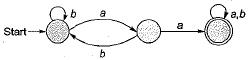First draw FSM for accepting all strings containing consecutive a’s, as shown above. Now change the final states to non final states and non final states to final states to get the required DFA shown below: